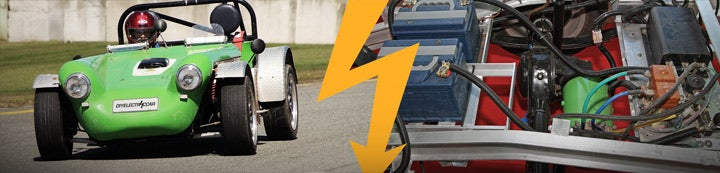1 - 4 of 4 Posts

#### BenNelson

·
##### Registered
Joined
·
203 Posts
Discussion Starter · ·
Hi All,

I have been told that it is pretty easy to convert a 12 volt volt meter to measure say a 36 or 48 volt system.

Appartently all one has to do is add a resistor inline.

I would like to gut the tachometer on my electric motorcycle and replace it with either a volt meter or amp meter.

If I used a volt meter like this one:
http://www.electricvehiclesusa.com/product_p/me-108772.htm

What would it take to make it measure a 36 or 48 volt battery pack? Does anyone know specifically what and how to hook a resistor to that to make it work?

#### Coley

·
##### Registered
Joined
·
1,369 Posts
I have a 50/100 volt meter that I wanted to run on the 100 volt side.
I was told to measure the resistance in the meter itself and put a matching resister across the two posts (+ & -).

Then see how close it was to the reading I wanted and adjust accordingly.
I don't know if it would work, because I bought a pair of Digital meters (volt & amp) from ZEVA.

They will fit better than my large voltmeter and I will have both readings.

#### rbgrn

·
##### Average Joe
Joined
·
705 Posts
Given Ohm's Law (P=IR) I think you need to know how much current the meter pulls at 12v to determine the correct resistance at 36 or 48. I'd just buy it (it's \$5!) check its resistance or current draw and calculate accordingly. Just look around for Ohm's Law and you'll find your formula.

#### Mr. Sharkey

·
##### Registered
Joined
·
207 Posts
Yes.

No.

Maybe.

Interestingly, "voltmeters" in the common configuration are not reading volts at all but are ammeters reading current. A common value for "full scale" on a "voltmeter" is 1 milliampere (.001A or 1mA). The current is limited by a series resistor of a value selected to give the meter the proper current at the desired full scale voltage.

For instance, if you had a 1mA meter movement, and the full scale reading was to be 12 volts, you'd insert a 1,2000 ohm reisitor in series with the meter (12v/.001=12000). For 24 volt full scale, 2,4000 ohms (24/.001=24000), etc.

To complicate your situation, the meter you have specified is an expanded-scale meter, that is, it doesn't read "0" (left hand peg) until the voltage reaches 8 volts. Full scale is 18 volts. Does that meter movement use a simple series resistor, and meter ballistics to make the expanded scale, or are there internal electronics to offset the meter's negative pole (-) to 8 volts? If the latter, you can pretty much forget trying to make it work on 36 or 48. If it's expanded by ballistics, then you need to insert the correct series resistance to raise it's indicating voltage.

As for the value of the resistor, well, that depends entirely on the sensitivity of the meter movement. If the meter is a 1mA movement, and already has a 1,8000 ohm internal resistor (18/.001=18000), then you'd have to add 36000 ohm resistor (18000+36000=54000) for 36 volt, or 54000 ohms for 48 volt.

If the meter sensitivity is not 1mA, then you'd have a little science project on your hands. Connect the meter up to 18 volts with a series milliammeter and measure the current, do the math, select the resistor.

Or you could simply get a 10,000 potentiometer and wire it up in series with the meter and adjust for the correct reading.

1 - 4 of 4 Posts